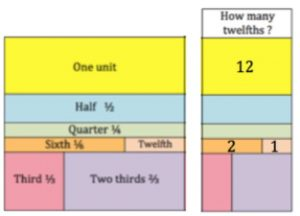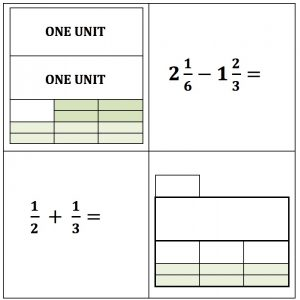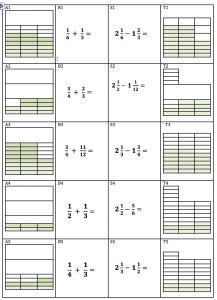Write the number of twelfths for each fraction to match the colours. Three of the answers are filled in.The answer to one of these calculations is $$\frac{1}{2}$$ and the other is $$\frac {5}{6}$$. Match one of the pictures to each calculation.Now for the big challenge. Can you sort these 20 cards into 5 sets matching each A card with a B, S and T card?

Each frame is 3 units.

You can  download the cards here then cut them out to sort them.